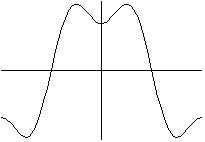Making a Graph with Maple

To graph the function y = cos(x) - (1/3)cos(3x) on the interval from -Pi to +Pi, you type this
> plot( cos(x) - (1/3)*cos(3*x), x = -Pi..Pi );

and you get this:The symbol > is the "prompt." Don't type it: the computer displays it when it is ready to receive your commands.

The graph above is a simple example of a Fourier series .

Back to Maple
Back to Department of Mathematics, University of Utah